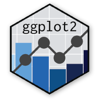Show Sidebar Hide Sidebar# geom_boxplot in ggplot2

How to make a box plot in ggplot2. Examples of box plots in R that are grouped, colored, and display the underlying data distribution.

### New to Plotly?

Plotly's R library is free and open source!
You can set up Plotly to work in online or offline mode.
We also have a quick-reference cheatsheet (new!) to help you get started!

### Version Check

Version 4 of Plotly's R package is now available!
Check out this post for more information on breaking changes and new features available in this version.

library(plotly)
packageVersion('plotly')

##  '4.9.1'


### Basic Boxplot

library(plotly)

set.seed(1234)
dat <- data.frame(cond = factor(rep(c("A","B"), each=200)), rating = c(rnorm(200),rnorm(200, mean=.8)))

p <- ggplot(dat, aes(x=cond, y=rating)) + geom_boxplot()

p <- ggplotly(p)

p


### Colored Boxplot

library(plotly)

set.seed(1234)
dat <- data.frame(cond = factor(rep(c("A","B"), each=200)), rating = c(rnorm(200),rnorm(200, mean=.8)))

p <- ggplot(dat, aes(x=cond, y=rating, fill=cond)) + geom_boxplot()

p <- ggplotly(p)

p


### Flipped Boxplot

library(plotly)

set.seed(1234)
dat <- data.frame(cond = factor(rep(c("A","B"), each=200)), rating = c(rnorm(200),rnorm(200, mean=.8)))

p <- ggplot(dat, aes(x=cond, y=rating, fill=cond)) + geom_boxplot() +
guides(fill=FALSE) + coord_flip()

p <- ggplotly(p)

p


### Boxplot w/ Stats

library(plotly)

set.seed(1234)
dat <- data.frame(cond = factor(rep(c("A","B"), each=200)), rating = c(rnorm(200),rnorm(200, mean=.8)))

p <- ggplot(dat, aes(x=cond, y=rating)) + geom_boxplot() +
stat_summary(fun.y=mean, geom="point", shape=5, size=4)

p <- ggplotly(p)

p


### Boxplot Facets

library(plyr)
library(reshape2)
library(plotly)

set.seed(1234)
x<- rnorm(100)
y.1<-rnorm(100)
y.2<-rnorm(100)
y.3<-rnorm(100)
y.4<-rnorm(100)

df<- (as.data.frame(cbind(x,y.1,y.2,y.3,y.4)))

dfmelt<-melt(df, measure.vars = 2:5)

p <- ggplot(dfmelt, aes(x=factor(round_any(x,0.5)), y=value,fill=variable))+
geom_boxplot()+
facet_grid(.~variable)+
labs(x="X (binned)")+
theme(axis.text.x=element_text(angle=-90, vjust=0.4,hjust=1))

p <- ggplotly(p)

p


### Time Series Facets

library(foreign)
library(MASS)
library(Hmisc)
library(reshape2)
library(plotly)

invisible(lapply(dat[, c("apply", "pared", "public")], table))
invisible(ftable(xtabs(~ public + apply + pared, data = dat)))

p <- ggplot(dat, aes(x = apply, y = gpa)) +
geom_boxplot(size = .75) +
facet_grid(pared ~ public, margins = TRUE)

p <- ggplotly(p)

p


### Outliers

library(plotly)
set.seed(123)

df <- diamonds[sample(1:nrow(diamonds), size = 1000),]

p <- ggplot(df, aes(cut, price, fill = cut)) +
geom_boxplot(outlier.shape = NA) +
ggtitle("Ignore outliers in ggplot2")

# Need to modify the plotly object and make outlier points have opacity equal to 0
p <- plotly_build(p)

p$data <- lapply(p$data, FUN = function(x){
x$marker = list(opacity = 0) return(x) }) p  ### Linewidth library(plotly) set.seed(123) df <- diamonds[sample(1:nrow(diamonds), size = 1000),] p <- ggplot(df, aes(cut, price, fill = cut)) + geom_boxplot(size = 1) + ggtitle("Adjust line width of boxplot in ggplot2") # Need to modify the plotly object to make sure line width is larger than default p <- plotly_build(p) p$data <- lapply(p$data, FUN = function(x){ x$line = list(width = 10)
return(x)
})

p


### Whiskers

library(plotly)
set.seed(123)

df <- diamonds[sample(1:nrow(diamonds), size = 1000),]

# This is how it needs to be done in ggplot
p <- ggplot(df, aes(color, price)) +
stat_boxplot(geom ='errorbar') +
geom_boxplot()+
ggtitle("Add horizontal lines to whiskers using ggplot2")

# Note that plotly will automatically add horozontal lines to the whiskers
p <- ggplot(df, aes(cut, price, fill = cut)) +
geom_boxplot()+
ggtitle("Add horizontal lines to whiskers using ggplot2")

p <- ggplotly(p)

p


These example were inspired by Cookbook for R.